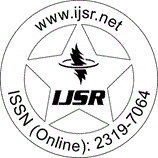International Journal of Science and Research (IJSR)
Call for Papers | Fully Refereed | Open Access | Double Blind Peer Reviewed

Research Paper | Mathematics | India | Volume 3 Issue 4, April 2014

# Multigrid Approach for Solving Elliptic Type Partial Differential Equations

Ashish Kumar Garg  | Itendra Kumar  | Sham Bansal  | Ishu Goyal 

Abstract: The present work is to develop numerical solutions for elliptic type partial differential equations using finite difference method. To establish this work we first present and classify the partial differential equations. Next we present and describe the multigrid methods. Applications of multigrid methods for the numerical solution of one-dimensional and two dimensional Poissons equation have been discussed. All the implementations have done by using Matlab.

Keywords: Partial differential equations, Finite difference method, Central difference, Multigrid methods, Poissons equation

Edition: Volume 3 Issue 4, April 2014,

Pages: 473 - 475